Courses

# Theories Of Mass Transfer Chemical Engineering Notes | EduRev

## Chemical Engineering : Theories Of Mass Transfer Chemical Engineering Notes | EduRev

The document Theories Of Mass Transfer Chemical Engineering Notes | EduRev is a part of the Chemical Engineering Course Mass Transfer.
All you need of Chemical Engineering at this link: Chemical Engineering

3.5 THEORIES OF MASS TRANSFER
Many theories are available to explain the behavior of mass transfer coefficient such as the film, penetration, surface renewal and others. The theories are based on both molecular and turbulent diffusion. The theories are explained in details in the following sections.

3.5.1 Film theory
In case of one dimensional convective mass transfer, the concentration distribution is linear. The coefficient of mass transfer in this special case is proportional to the diffusion coefficient and inversely proportional to the film. This phenomenon is commonly known as the film theory. The schematic diagram of concentration profile with stagnant film is shown in Figure 3.3.
The expression of mass transfer coefficient is developed theoretically by visualizing the mechanism. This is done by film theory. Film theory is based on the following assumptions:
a) Mass transfer occurs by molecular diffusion through a fluid layer at phase boundary (solid wall). Beyond this film, concentration is homogeneous and is CAb.
b) Mass transfer through the film occurs at steady state.
c) Flux is low and mass transfer occurs at low concentration.
Hence,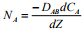(3.51)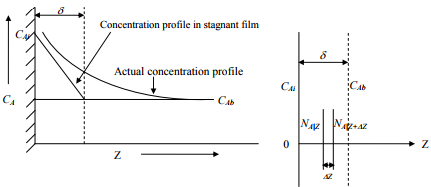3.3: Schematic diagram of concentration profile with stagnant film.

Steady-state mass balance is done over an elementary volume of thickness ΔZ.
Rate of input of solute at Z=NA|Z
Rate of output of solute at Z+ΔZ=NA|Z+ΔZ
Rate of accumulation=0= (rate of input-rate of output) Therefore, at steady state, NA|Z-NA|Z+ΔZ=0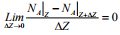(3.52)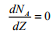(3.53)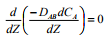(3.54)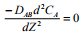(3.55)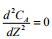(3.56)
Integrating Equation (3.55) for the following boundary conditions:
CA=CAi when Z=0
CA=CAb when Z=δ
We have now,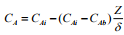Hence, according to film theory, concentration profile in stagnant film is linear. Molar flux through film, NA: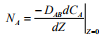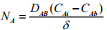(3.58)
Now comparing Equation (3.58) with Equation (3.8),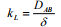(3.59)
Film theory is useful in the analysis of mass transfer with chemical reaction. For turbulent flow, the mass transfer coefficient has much smaller dependency compared to laminar flow. In the turbulent flow, the mass transfer coefficient is proportional to n DnAB where n may be zero to 0.9, depending upon the operating conditions. Although the film theory offers some explanation of the mechanism of mass transfer in fluid media, it does not explain the estimation thickness of the film. Due to this disadvantage, application of the model is restricted to mass transfer in a diffusion cell.

Offer running on EduRev: Apply code STAYHOME200 to get INR 200 off on our premium plan EduRev Infinity!

## Mass Transfer

29 videos|45 docs|44 tests

,

,

,

,

,

,

,

,

,

,

,

,

,

,

,

,

,

,

,

,

,

;